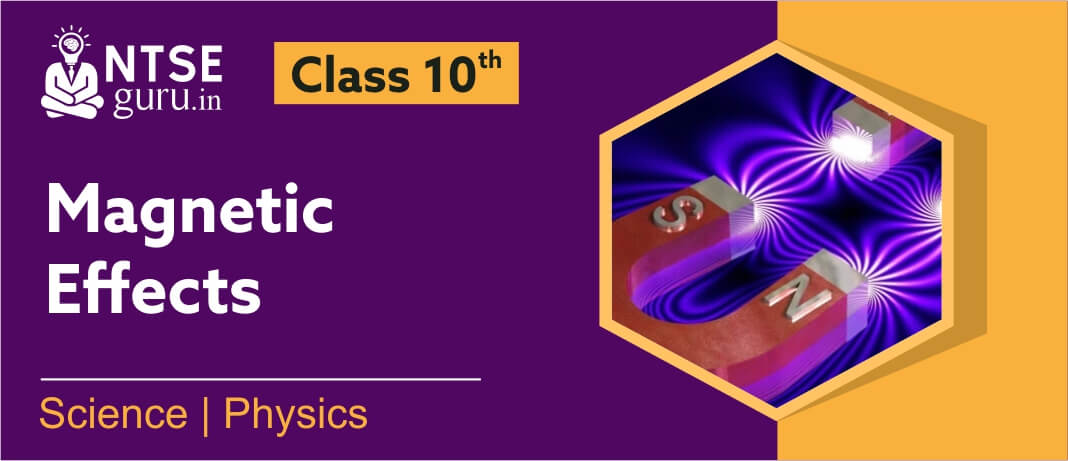# Magnetic Effects Of Electric Current Class 10Board exams for class X are around the corner and now it’s high time to revise all the important concepts and related questions so as to score well. When it comes to Physics, it becomes very important to go through the basic concepts and Various Formulae’s and their application in our daily lives.

Class 10 Science Chapter 13 in NCERT Science deals with Various Effects of Electric Current in detail. The chapter, Magnetic Effects of Electric Current Class 10 includes concepts of Magnetism, Magnetic field due to current carrying conductor, Circular Loop and solenoid, Right hand Thumb Rule, Fleming’s Left hand Rule. It also includes important practical topics like Electric Generator, Electric Motor, Electromagnetic Induction detailed insight of Domestic Electric Circuits and their application in real-life situations are included in the chapter.

## Class 10 Science Chapter 10 - Important Questions

1. Does a magnetic field exert force on charge particle? If yes, give the expression for the same.

Ans. In a magnetic field, a moving charged particle experiences a force (F).

$$F=Bqv\sin \theta$$

Here     B =       strength of magnetic field.

q  =      charge present on particle.

$$v$$ =       velocity of the charge particle.

$$\theta$$ =        angle between the direction of motion of charge particle

and the direction of magnetic field

2. A charged particle enters into a magnetic field such that its velocity & direction of magnetic field are mutually perpendicular to each other. Predict the locus of that charged particle. Also State the impact of magnetic field on the velocity of that charged particle.

Ans. When a charged particle is projected perpendicular to the magnetic field then its path will be circular in a plane perpendicular to the plane of magnetic field and the direction of motion of charged particle. Magnitude of its velocity will be constant.

3. What are the advantages of A.C over D.C & vice versa?

Ans. Advantages of AC over DC are as follows:

(i)   AC can be transmitted over long distance without much loss of energy & with low cost of transmission. DC cannot be transmitted as such.

(ii)  AC voltage can be easily varied by using transformer.

(iii) AC can be easily converted into DC.

(iv) AC is easier & cheaper to generate than DC.

(v)  AC equipments are very durable & hence do not need much maintenance.

Advantages of D.C. over A.C. are as follows:

(i)   It is more dangerous to work with A.C. at high voltage than D.C.

(ii)  AC cannot be used for electroplating, electro refining & other electrolytic processes. In such processes DC is required.

4. On what factor does the value of induced EMF in a coil depend? A copper coil and an iron coil of same dimensions are subjected to same changes of magnetic flux per unit time. Will they produce same EMF & induced current or they differ?

Ans. Value of induced EMF in a coil depends upon the following factors:

(i)  Change in magnetic field at the location of coil

(ii) Rotation of the coil in a uniform magnetic field.

(iii) Change in area of coil in the magnetic field.

Both produce same induced EMF but induced current will be different.

5. Why all domestic appliances are connected in parallel & not in series combination?

Ans. In domestic circuit, appliances are connected in parallel because,

(i)   All appliances have same voltage so, work efficiently.

(ii)  If anyone appliance is switched off or damaged then remaining devices remain unaffected & continue to work.

(iii) In series arrangement, all the appliances will work simultaneously whether we need or need not but in parallel we can operate them independently with the help of switch.

NTSEguru helps you to boost your preparation with the most probable questions of the chapter Magentic Effects of Electric Current Class 10  in accordance with the revised pattern of X board examination by our expert team of faculties.

Have a great time learning with us!